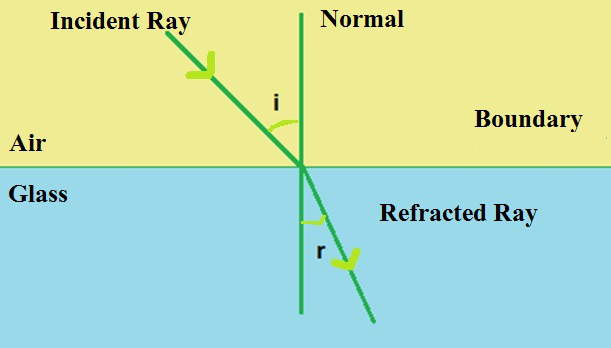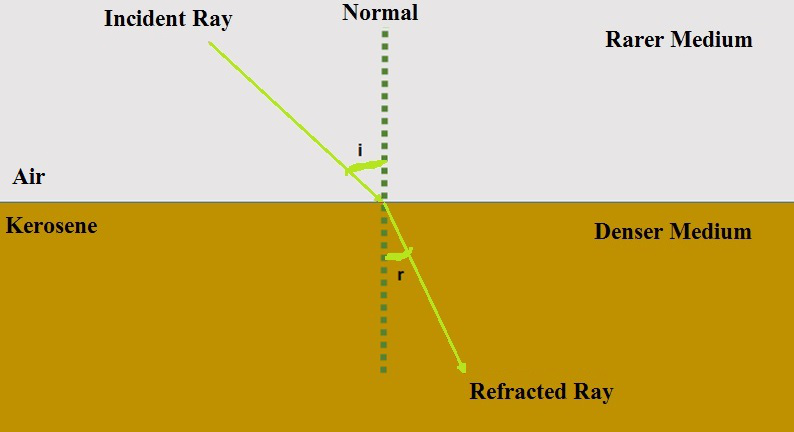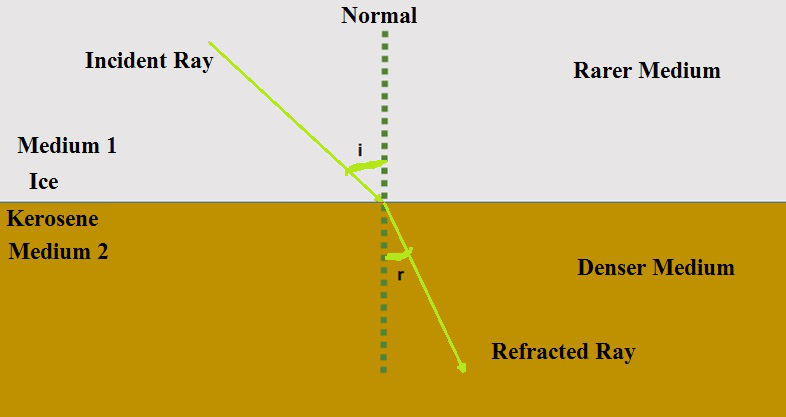Related Articles

# Refractive Index

• Last Updated : 30 Apr, 2021

By watching some very common optical phenomena that surround or occurs around us, we may conclude to the point that the light rays seem to travel in straight lines. Sometime in the twentieth era, the popular wave theory of light often became slightly inadequate or irrelevant for cases of the interaction of light with matter, and light often behaves somewhat or closely like a stream or system of particles. This discussion and arguments about the true nature of light continued for years-long till a modern quantum theory of light came, finally in which light is neither having a ‘wave’ nor a ‘particle’ in nature – the new theory intends to relate the particle properties of light with the wave nature of it. The refraction of light travelling through different media is explained briefly and easily using the straight-line propagation of light rays in this article.

### Refraction of Light

It sometimes appears or observed that when the light rays are travelling obliquely from one medium to another medium, the path or direction of the propagation of light in another or second medium somehow changes. This certain phenomenon is what is known as the refraction of light. It is in very simple words describes the change in the velocity or speed of light when it goes or travels from one medium to another medium. The refraction of light depends upon the velocity of the material medium we use and the nature of another medium from which the light comes. There are certain laws for it also which this refraction phenomenon follows. The observable change in the velocity or speed of light rays causes refraction.Refraction through two different media or mediums is shown above. The light ray has changed its path on travelling from air to glass, and it will again change when vice versa occurs.

### Laws Of Refraction

There are given two laws of refraction as stated below which at the sight of refraction the light follows and what we see is the refracted image formed of the object.

• The refracted ray, incident or what we call obliquely falling ray and the normal ray at the point of incidence all will tend to lie together in the same plane.
• Secondly, we have the ratio of sin of the angle of the incidence and refraction is a constant or has a definite value which is stated or termed as Snell’s law.
•= constant
• Where we have i = angle of incidence, r = angle of refraction, the constant value which depends upon the refractive indexes of the two taken mediums. It is their ratio and is dimensionless.

### Refractive Index

Refractive Index first of all is a dimensionless quantity. Index of refraction or in other words refractive index enables us to know how fast light travels through the material medium. The refractive index gives an idea about the speed of light while travelling in a different medium. Whenever the light that tends to travel obliquely from one medium to another changes its direction while travelling from another, the extent of change in the direction of light rays is what we say and calculate as refractive index. The ratio of the velocities or speed of light in different media gives the refractive index.

The refractive index is of two types:

1. Absolute Refractive Index
2. Relative Refractive Index

The type of refractive index depends upon the two mediums in which the light is traveling. The absolute refractive index has one material medium and one is vacuum in which the speed of light is 3 × 108 m/s. The relative refractive index is the relative change in speed or velocity of light upon travelling from one given medium to another.

Upon travelling from a rarer medium to denser the light rays tend to bend towards the normal and if it travels from denser to rarer then it bends away from the normal at the point of incidence.

### Absolute Refractive Index

For a considered material medium, the refractive index is observed or considered to be the ratio between the speed or velocity of light in a vacuum (c) to the speed of light in the provided material medium (v) on which it falls. Refractive index or index of refraction for a medium is represented or denoted by small n, and it is given by the following formula:Where c = speed or velocity of the light in vacuum, v = speed or velocity of light in the provided medium.

The speed or what we say the velocity of light in a vacuum is 3 × 108 m/s. Its speed in air is also almost the same as that in vacuum with a minimal difference. Hence, when travelling from air to the medium the speed is taken to be 3 × 108 m/s only. The absolute refractive index as the name suggests gives us a rough estimate of the optical density of the given material.

The following table gives an idea about different refractive index of mediums. The one with a higher refractive index is optically denser than a material with a low refractive index which becomes a rarer medium. A material having a higher optical density doesn’t mean to have a high mass by volume density as they are two different quantities. For instance, kerosene has a lower density than water, but it is optically denser than water as we can see it has a higher value of the refractive index of 1.44 than water 1.33.### Applications

• Gives an idea about the absolute refractive index of many material mediums other than vacuum and air so that we can use it for performing laboratory activities.
• Is used in industries to make certain chemicals with the known refractive indexes of the materials.
• They are used in pharmaceutical industries to provide a rough estimate of the optical densities of the chemicals.
• The values of the absolute refractive index are widely used to differentiate optically denser and rarer mediums.

### Relative Refractive Index

The relative refractive index refers to the refractive index of one material medium with respect to another one. The given velocities of light in different media can give the relative refractive index by the following also where the first medium is not vacuum:The n21 = refractive index of the speed of light in material medium 2 with respect to the speed or velocity of light in medium 1.

Similarly, we can also calculate the refractive index of 1 with respect to 2. By the ratio of the velocity of light in medium 2 with respect to the velocity of light in medium 1.On travelling from a rarer to denser medium, light ray bends towards normal and vice versa, that on travelling from denser to rarer it bends away from normal. As it can be observed and seen that the refractive index of ice is lower than that of kerosene so the light ray after travelling from ice to kerosene has bent towards the normal and so their ratio can give us the relative refractive index.### Applications

• It is widely used or applied for identifying a particular substance, confirm its purity, or measure the given concentration.
• Generally and commonly it is used for measuring the concentration of the solute in an aqueous solution. For instance, a solution of sugar, the refractive index of it can be used to determine the sugar content.
• It can also be used also in the determination of the drug concentration in the pharmaceutical or pharmacy industry.
• It is widely used to calculate the focusing power of various lenses, and the dispersive power of the prisms.
• Also, it is generally applied for the estimation of the thermophysical properties of many hydrocarbons and of petroleum mixtures.

### Sample Questions

Question 1: What is the constant value if the angle of refraction is given to be 15° and the angle of incidence is 32°?

Solution:

As we know,=constant

Given sin i= sin 32° and sin r= sin 15°

Putting the respective values of the given angles from log table we get= 0.84

Hence, the constant for the above given values is 0.84.

Question 2: What is the value of the sin of the angle of incidence if the angle of refraction is given to be 35°? The constant is assumed to be 1.34.

Solution:

As we know,=constant

Given constant has value = 1.34 and sin r = sin 35° = 0.57

Putting the respective values of the angles from log table we getTherefore, sin i = 1.34 × 0.57

Therefore, sin i = 0.763

Hence, sin of angle of incidence or sin i = 0.763.

Question 3: What is the constant value if the angle of incidence is 45° and the angle of refraction is given to be 30°?

Solution:

As we know,=constant

Given sin i = sin 45° and sin r = sin 30°

Putting the respective values of the given angles from log table we getHence, the value of the constant is found to be 1.44.

Question 4: Calculate the speed of light in water and also in ice. The absolute refractive index of ice is 1.31 and water is 1.53. In which medium is the speed highest?

Solution:

As we know,Where refractive index of water n= 1.53, c = 3 × 108 m/sHence, the velocity or speed of light in water is vw​ = 1.96 × 108 m/s.

In the second case, where refractive index of ice n = 1.31, c = 3 × 108 m/sHence, the velocity or speed of light in ice is vi​ = 2.29 × 108 m/s.

Therefore, the velocity of light is greater in case of ice than in water.

Question 5: Calculate the speed of light in benzene. The absolute refractive index of benzene is 1.50.

Solution:

As we know we can calculate the refractive index by the following formula,Where refractive index of benzene n= 1.50, c = 3 × 108 m/sHence, the velocity or speed of light in kerosene is vb​ = 2 × 108 m/s

Question 6: The velocity of light in kerosene is 2.08 × 108 m/s and in water is 1.96 × 108 m/s. By referring the given values calculate or find the refractive index of the kerosene with respect to water medium.

Solution:

As we know,Given,m/s.Hence the refractive index ratio of kerosene in respect to second medium water is 0.94.

Question 7: The velocity of light in glass is 1.97 × 108 m/s and in water is 1.96 × 108 m/s. By referring to the above calculate or find the refractive index of water with respect to glass.

Solution:

As we know,Given,m/s.Hence the refractive index of glass with respect to water is 1.005.

Question 8: The velocity of light in kerosene is 2.08 × 108 m/s and in glass is 1.97 × 108 m/s. Calculate the refractive index of glass with respect to kerosene.

Solution:

As we know,Given,m/s,m/s.Hence, the refractive index of glass with respect to kerosene is 1.05.

Attention reader! All those who say programming isn’t for kids, just haven’t met the right mentors yet. Join the  Demo Class for First Step to Coding Course, specifically designed for students of class 8 to 12.

The students will get to learn more about the world of programming in these free classes which will definitely help them in making a wise career choice in the future.

My Personal Notes arrow_drop_up